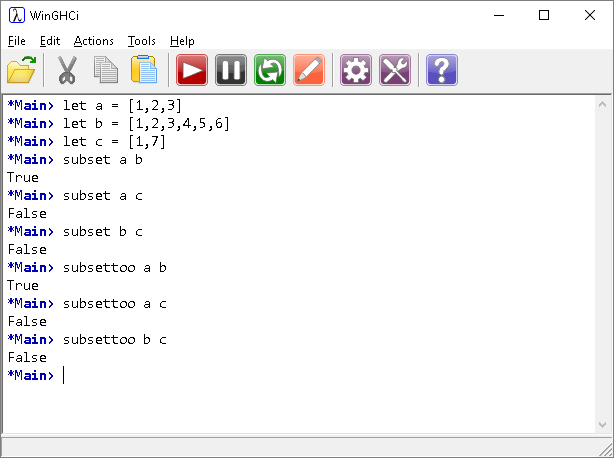# Introduction To Haskell Checking Subsets

## Introduction

There are modules with this functionality but it''s a nice way to think about the language. Here are two functions that test with set a is a subset of set b.

```subset a b = null [x | x<-a ,elem x b == False]
subsettoo a b = all (`elem` b) a
```

The first version uses a list comprehension to make a list of items that from set a which are not in set b. If this list is empty, then a is a subset of b.

The second version uses the all function to test if the predicate (in brackets) is true of all elements in the list. In this case it checks if all members of set a are in set b. Notice the use of the grave quotes to turn a prefix function into an infix function.## Challenges

1. There are some other ways to do the subset checking. See if you can think of them (eg filtering).
2. Although there are readily available libraries with all of the functionality for working with sets, it's worth having a go at implementing the basics by yourself. Intersection, union and difference are the operations that you want to work out.